Main Module 4 Comparing Multiple Samples of Numerical Data

 Learning Objectives At the end of the module, the student will: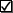Understand the concept and objective of two-sample tests with numerical data.Using Microsoft Excel, select, perform and interpret the: Appropriate hypothesis test for the difference between two means based on samples drawn from independent populations. Hypothesis test for the difference between two variances based on samples drawn from independent populations. Hypothesis test for the difference between two means based on samples drawn from related populations. Wilcoxon Rank Sum Nonparametric Test for differences in two medians.Understand the concept of and objective of multiple sample tests with numerical data.Using Microsoft Excel, select, perform and interpret the: Hypothesis test for differences in multiple means in one-way completely randomized ANOVA model. Bonferroni Multiple Comparisons Procedure to determine which of multiple means are different in a one-way ANOVA. Kruskal-Wallis Rank Nonparametric Test for differences in multiple medians. Hypothesis test for differences in multiple means and for interaction in the two-factor factorial ANOVA model.
 Module Notes The following sub modules contain summary notes for the six content topic areas of Module 4.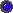Module 4.1: Comparing Two Independent Samples of Numerical DataModule 4.2: Comparing Two Related Samples of Numerical DataModule 4.3: Comparing Two Samples Using a Nonparametric TestModule 4.4: Comparing Multiple Samples of Numerical Data: One FactorModule 4.5: Comparing Multiple Samples of Numerical Data: Two FactorsModule 4.6: Comparing Multiple Samples of Numerical Data: A Nonparametric Test Assignment Reference the data in the Table of Product Ratings at the bottom of this page. These are product ratings from randomly selected test market participants using a new product after viewing five different product advertisements. For example, the new product was rated a 15 by the first participant viewing Advertisement A. The rating scale is numeric with a range of 0 to 20, 20 being the highest rating. This optional exercise requires that you do the Excel analysis. This is good practice and helps prepare you to use this technique in the future. However, for the final exam, I will provide the Excel data analysis input/output so the exam concentrates on your interpretations and conclusions from the Excel input/output. This assignment is not for turn-in/grading. I will provide Excel printouts and answers to the following questions via e-mail attachment so you do not have to develop the Excel Worksheets - just interpret them. Using Microsoft Excel for the analysis, 1. Enter the data in a new spreadsheet. 2. Select, perform and interpret the appropriate t-test for the difference between two means. Compare the sample in Colume E in the Table of Product Ratings for Five Advertisements; against the sample of New Data (from high school students). Hint: run an F-test for differences between two variances to determine which t-test to use. 3. Use the Wilcoxon Rank Sum Test to determine if there is a difference in the two medians in the data identified in Item 2. Is your conclusion to this test the same as that of the t-test in Item 2 with respect to rejecting or failing to reject the null hypothesis of equality? 4. Perform and interpret the one-way ANOVA for differences in multiple means using the data in the table of Product Ratings for Five Advertisements. If you determine that there is a significant difference in the five means, use the Bonferroni Multiple Comparison Procedure to determine which pairs of means are different. 5. Perform and interpret the Kruskal-Walis Rank Test for differences in multiple medians using the data in the table of Product Ratings for Five Advertisements. Is your conclusion to this test the same as that of the one-way ANOVA in Item 4 with respect to rejecting or failing to reject the null hypothesis of equality? 6. Now assume the first three rows of the data in the table of Product Ratings for Five Advertisements are responses from men and the last three rows are responses from women. Use a two-factor ANOVA (with replication) to determine if there is a significant interaction effect, and if not, if the gender and/or advertising group effects are significant (means are not equal). 7. Be able to demonstrate your knowledge of the learning objectives, as applied to this Assignment, in Exam 4. 8. (Optional). Send Microsoft Excel file, with Items 1 - 6 as an attachment to an e-mail to the instructor if you wish the instructor to review/give feedback on your work. This should be done not later than November 17, 2001. Optional Text Reading Anderson, D., Sweeney, D., & Williams, T. (2001). Contemporary Business Statistics with Microsoft Excel. Cincinnati, OH: South-Western. Chapter 10

Table of Product Ratings

 A B C D E New Data 15 16 8 5 12 14 18 17 7 6 19 13 17 21 10 13 18 15 19 16 15 11 12 9 19 19 14 9 17 11 20 17 14 10 14 13 12 16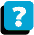About the Course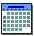Module Schedule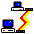WebBoard